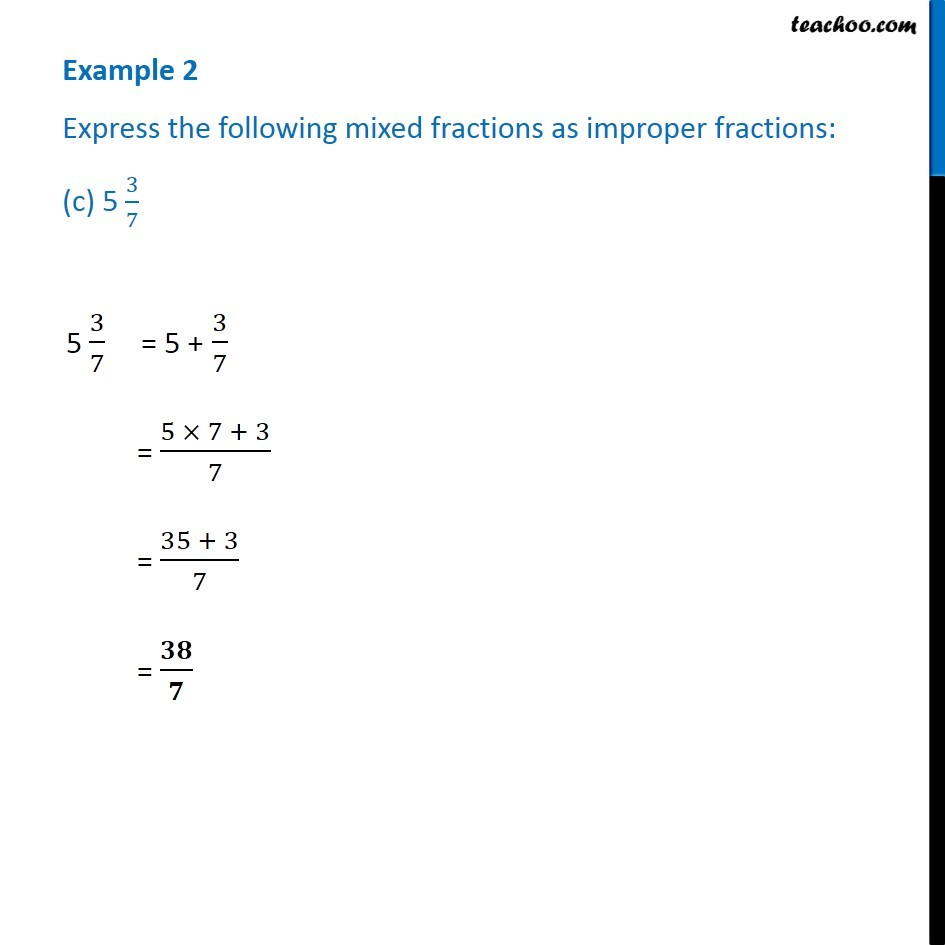Examples

Chapter 7 Class 6 Fractions
Serial order wiseLearn in your speed, with individual attention - Teachoo Maths 1-on-1 Class

### Transcript

Example 2 Express the following mixed fractions as improper fractions: (c) 5 3/7 5 3/7 = 5 + 3/7 = (5 × 7 + 3)/7 = (35 + 3)/7 = 𝟑𝟖/𝟕